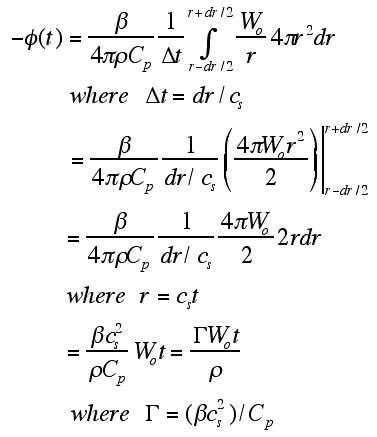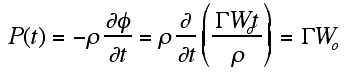# The Mathematics

This page illustrates the computation using discretized computer simulation. Consider a computational time step Δt [s] and a position stepsize dr [m].

Consider the pressure observed at the center of a sphere in which energy is deposited uniformly at time zero. The velocity potential φ at time t would be proportional to the energy deposition Wo deposited in a spherical shell at a distance r = cst from the center. There is also a 1/r dependence to φ. For this simple example, a simple expression describes the time-resolved velocity potential:The pressure is related to the time derivative of φ:The convention describes -φ as proportional to Wo, and P as proportional to -dφ/dt, so P is proportional to Wo.

IN SUMMARY, the pressue P is proportional to GWo, where Γ is called the Grueneisen coefficient, a dimensionless factor that describes the fraction of thermal energy deposition [J/m3] that converts to pressure [J/m3]. This holds true until time t = radius/cs where radius is the radius of the sphere. At times after this, there is no velocity potential because there is no Wo deposited beyond the sphere. φ goes to zero which is constant, so pressure goes to zero too.